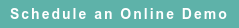# RoyaltyStat Blog

### Posts by Topic

Innovate, innovate, says Schumpeter and his prophets, because innovation is associated with cost reduction and increased profits. However, many controlled (within group) corporate reorganizations lack economic substance because they violate this basic principle of innovation. Ergo, an objective of innovation is to reduce average costs and therefore to increase profits.  We provide a theorem and include an arithmetic proof that cost-reducing innovations (holding the product or service price constant) increase profits.

Assume that a given product or service price is a function of time, P(t), and that price is proportional to average cost C(t) via the markup equation:

(1)     P(t) = m(t) C(t), where m(t) > 1 is the profit markup at time t.

Assume also a cost-reducing innovation such that the cost of the product at time t2, namely C(t2), is smaller than the cost at a previous time (t2 > t1):

(2)     C(t1) > C(t2).

Thus, from (1) and (2), we can posit the theorem:

If P(t1) = m(t1) C(t1) and P(t2) = m(t2) C(t2) with C(t1) > C(t2) and P(t1) = P(t2), then it follows that m(t2) > m(t1).

This theorem is read as follows: corporate reorganizations and other predictable business innovations are aimed to decrease costs with time (otherwise innovations make no economic sense), and while the product or service price remains constant before and after the innovation, imply that the profit markup is increased with time.

This means that controlled taxpayers have a heavy burden to establish that after a controlled reorganization profits decrease instead of the expected increase. In this regard, outsourced business functions (such as stripping a controlled distributor or retailer of procurement or ancillary service functions) make sense only if m(t2) > m(t1), that is, the profit markup is higher and not lower after the corporate reorganziation of economic activities.

Proof that cost-reducing innovations lead to higher profits:

(3)     P(t1) = P(t2) implies that m(t1) C(t1) = m(t2) C(t2) or

(4)     (C(t1) / C(t2)) = (m(t2) / m(t1)).

The cost-reducing innovation assumption (C(t1) > C(t2)) which implies that:

(5)     (C(t1) / C(t2)) > 1,

since from equation (4) we have the result that (m(t2) / m(t1)) = (C(t1) / C(t2)).

It follows from equations (4) and (5) that:

(6)     (m(t2) / m(t1)) > 1 and thus m(t2) > m(t1).

Quod Erat Demonstrandum (QED)

Published on Oct 15, 2019 3:38:51 PM

Ednaldo Silva (Ph.D.) is founder and managing director of RoyaltyStat. He helped draft the US transfer pricing regulations and developed the comparable profits method called TNNM by the OECD. He can be contacted at: esilva@royaltystat.com

RoyaltyStat provides premier online databases of royalty rates extracted from unredacted license agreements
and normalized company financials (income statement, balance sheet, cash flow). We provide high-quality data, built-in analytical tools, customer training and attentive technical support.Topics: Economic Substance